December 5th , 2008

J.H.Grosch, K.Lipkin, J.Ozer

## Introduction

• Electromagnetic suspension (EMS), which uses the electromagnetic attractive force of the magnets to lift up a train from the rails.
• Electrodynamic suspension (EDS), which uses the repulsive forces between two magnets to lift up the train from the rail.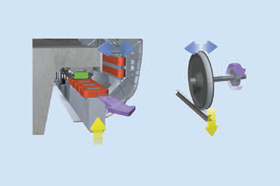Figure 1: A Maglev Train Compared to a Conventional Wheel

Our group chose the EMS system because it is unstable by nature, which makes for a more interesting controller design.

For this system, it is necessary to keep the separation between the rail and the train in a constant range. A common value for this distance is about 10 mm. We will develop the control using a coupled electro-mechanical model to get a realistic impression of the settings.Figure 2: Front View of a Maglev Train on a Track

Nevertheless there are many problems in realization of magnetic transportation systems. The biggest problem is the requirement for expensive new infrastructure like new rail systems. By contrast, conventional High Speed Trains such as the French TGV can use existing rail structure. Nevertheless the impressive and new developed maglev technology causes many different realization studies worldwide for maglev transportation, even in North America.

For example there is a regional study for a realization of a “Transrapid” in Pittsburgh with a 90 km length of the pipe. The roadway arrangement may be by the Airport via Downtown to Greensburg.  The investment volume is calculated as about 3.5 billion US\$. The goal of this train should be the relief of the Pittsburgh traffic. An additional possibility could be the upgrading to new maglev rails on the east coast between Boston and Washington DC.

# Tasks and Objective

The main idea of our project is to design a controller to control the distance of a magnetic levitation train and the rail. At the beginning there are only the two main forces included in the model – gravity and magnetic attraction. Additionally there can be some other disturbance forces in the end model. In the first step we defined the mathematical model describing the system. Therefore we determined the Transfer Function from a mechanical and an electrical model, which are both connected in each other.  Based on these models the Simulink Model was formed.

In the next step we had to take a closer look on the structure of the controller. We found that we needed a number of integrators, derivative controllers, lead compensators, and gains to successfully control the system.  Our controller ended up meeting all of our design specifications.

# Mathematical Model for System

We chose to model a Magnetic Levitation train.  The system both mechanical and electrical components.

### Assumptions and Knowns

In order to simulate a maglev train, we needed a train design.  Actual specifications for current maglev designs are not widely available, but based on partial specifications scattered throughout the web, and specs from wheeled commuter trains where necessary, we constructed our “model” train.

#### Assumptions

1. The equation for the attraction of an electromagnet to a piece of iron (in this case the track), is a math problem of sufficient difficulty as to be the subject of undergraduate and graduate research projects in physics.  However, since the magnetic field is proportional to the square of the current, and inversely proportional to the square of the distance of measure, it is safe to assume that the magnetic force takes the following form:

Where C is a constant.  The easiest way to accurately find this constant would be to experiment with the actual system.  In the case of our model, we found it by using other known properties of the system.  The derivation is in the “Deriving State Equations” section.  It is important to stress that this is not the actual, analytical model for magnetic force, simply a simplification that is relatively accurate when the deviations from the starting current and displacement are small.

2. There are two inputs into the system.  The first is the control input of voltage into the magnet.  The voltage controls the current, which controls the magnetic force.  The second input is a disturbance input of vertical force put onto the train.  In this report, we simulated this force as a person stepping onto the train at t=0.  The person weighs 150lb, or about 670 N.

3. The only forces on the train are vertical.  In a real maglev train, there would be multiple magnets located over the bottom to help counter moments the train may experience.  In order to simulate the train as a single degree of freedom system, the train may not experience any moments, and the only displacement is in the vertical direction.  When we refer to velocity or acceleration, unless otherwise noted, these are the vertical velocities and accelerations.

4. Several of our state equations were non-linear.  For the purposes of controller design, we created linear models of these equations to allow for state space evaluation.  All linearizations were accomplished using a first degree Taylor expansion of the following form:

All equations are linearized around the difference between the appropriate variable and its initial value.  For instance, voltage is linearized as δe, or (e-e0).  This is done so that we can ignore the initial conditions in our transfer function.

5. We needed initial conditions for our model.  In order to determine these, we referenced a paper on an experimental maglev train in Birmingham, England, that actually contained some of the technical information on their system.  They used a levitation of 10mm, and a 600V electrical system.  From these specs, we determined the following initial conditions:

These conditions result in a magnetic force equal to the weight of the train.

6. The magnet is a round magnet 10cm deep and two square meters in area.  We were unable to find much data on the magnet designs for commercial maglev trains.  Instead, we made a reasonable guess of the size of the magnet.  If the magnet is too small, the magnetic field would be so high as to rip the core apart.  Most maglev trains probably use many smaller magnets instead of one large one, but for the sake of simplicity, we used only one magnet.

7. The wire coil for the magnet is made out of AWG 000 aluminum solid core wire.  All properties of the wire are taken from the AWG specifications.  Aluminum wire was used because this is what was used in the Birmingham train we cited for many of our physical properties.  Copper or a super conductor would be much more appropriate.

## Identifying the State Variables

When setting up our state space matrices, we identify the energy storage elements as the kinetic and potential energy of the train mass (functions of v and x, respectively) and the inductance of the magnet (a function of i and x).  Thus, our state variables for this system are x, v, and i.  Solving for dx/dt is trivial and is simply equal to v by definition.  Equations for dv/dt and di/dt had to be derived by summing forces and solving for the current present in the circuit.  The inputs to the system are e, the voltage supplied to the circuit, and Fd, the disturbance force applied to the train which our system must stabilize.

The fact that we have 3 state variables leads us to believe that the transfer function will contain an s^3 term in the denominator.

## Deriving the State Equations

### The Mechanical System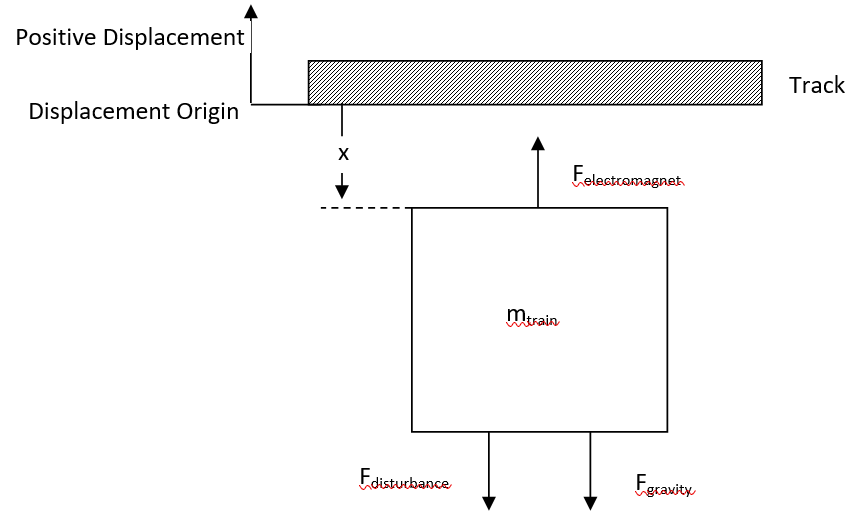Figure 4: Free Body Diagram of a Maglev Train

The sum of forces for this system is:

In order to develop a mathematical model, we can re-express the equation as such:

We will consider Fd­ later in the report.  First, we wanted to determine the unknown constant C.  Ideally, this constant might be determined using the very complicated physics of magnetic field.  In reality, this constant would be best determined by experimentation.  We determined it using our known properties of the system:

Since m, i, and x are known at the initial condition, we can determine that C = 1.2 x 10-4 Nm2A-2

The second state equation can be found simply:

### The Electrical System

The electrical system of the train is composed of a large ferrite core electromagnet.  The magnet’s properties are discussed in the “Assumptions” section.  We simulated our magnet with a constant voltage, a coil resistance, and an impedance.

Using Kirchoff’s loop rule, we can find the voltage as follows:

Where the final term is the back EMF caused by the large iron rail moving through the magnet’s field.

This can be rewritten to find the quantity of interest, di/dt:

### Linearization

Both our current and velocity equations are non-linear.  In order to evaluate them using a second order system, we had to linearize both of them.  To do this, we used the first term of the Taylor series expansion of each equation as described in our assumptions.  The linearized variables are perturbations about an operating point x0 = -0.01m, v0 = 0 m/s, i0 = 588 amps, and e0 = 600V.  These starting points are based upon the Birmingham Maglev train we found in our research (see Pollard in bibliography).

From our displacement system equation, we take the Taylor expansion.

Simplifying the Taylor expansion gives the following equation:

We converted the system from using initial conditions to using perturbations around the initial conditions.

Our final result:

We then linearized the current equation.

The 1st order Taylor expansion yields:

This simplifies to:

We again converted the equation to use perturbation variables in the same manner as the displacement equation.  This yielded the final result of:

### Transfer Function Evaluation

In order to evaluate the response of the system, we have to use Laplace transforms to bring the two equations into the frequency domain.  When we took the Laplace transform, we consider L(dx) – X(s) and so forth, instead of the more technically correct L(dx) – dX(s), for the sake of simplicity of notation.

Since vo = 0, we can simplify to:

Solving for I(S):

Now, we take the Laplace transform of the displacement equation:

Moving all the X(s) terms to one side gives us:

Substituting in the equation for I(s) we determined earlier, we get:

Simplify:

Gather all X(s) terms on the left hand side of the equation:

Divide both sides of the equation by the coefficient of X(s).

Simplify:

Expand the denominator:

For the F(s) coefficient, multiply both the numerator and denominator by (s+R/L) to get a regular polynomial:

Our final transfer function from our inputs of voltage and the disturbance force is:

## System Response

First we evaluated the step response of the system.  The step response was evaluated for each input separately.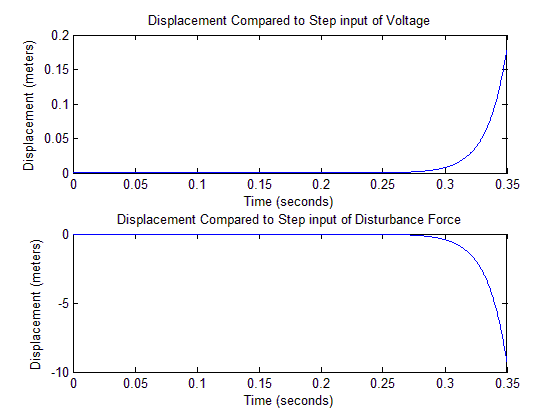Figure 6: Step Response of Linear Model for Voltage (top) and Disturbance (bottom)

These step inputs are for the perturbation inputs, so, a 1V step input means that the voltage is increased from v0to v0+1, in this case, from 600V to 601V.  The disturbance force is also a perturbation input, but since F0 = 0, δF = F.

Our results were as expected.  Increasing the voltage in the magnets increased the magnetic force, causing the train to rise towards the track.  Putting a disturbance force on the train, pushing downwards, cased the train to sink down.  Both responses grow exponentially, as the train accelerates from the magnetic force, in the case of a voltage step, or from the force of gravity, if the train receives a force step input.  The system is unstable with regards to both transfer functions.

We then used MATLAB to calculate the poles and zeros of our system.  Since we have two inputs, we calculated the poles and zeros for each transfer function separately.Figure 7: Polces and Zeros of Linear Model Transfer Function

The top graph shows the poles and zeros for the transfer function from E(s) to X(s).  The lower graph shows the transfer function from F(s) to X(s).  As expected, the pole zero plots show that both systems are unstable, since they have a pole in the right half plane.  The poles and zeros that appear to be at the origin are not actually zero, but have very small negative real values.  In the force transfer function, since the pole and zero are so close together, they will probably cancel each other out.

To test our potential controllers, we created two Simulink system models.  One system used our linearlized system equations.  This system is only accurate for very small perturbations from our initial conditions, but gives us the information we need to construct a controller.

Our second model uses the actual, non-linear system equations.  This system is much more accurate over a wider range of conditions than our linear system.  We will use the non-linear model for testing our controller, to get a better idea of how it would perform in the real world.

### Control goals

Our desired system behavior should control the distance between rail and train in a constant range, even with changing disturbances. Problems of too less distance would cause the clash of the maglev and the magnets of the rail.  In return too much distance would lead to an overshoot of the system.

For the designing of the controller we defined a set of main criteria which our controller should achieve.

1. The controller has to regulate the distance between train and rail on a constant range of 10 mm. It’s already described how changing in distances would influence the train behavior.
2. The overshoot of the system should be less than 50% of reference – so 5mm. There is only 10mm between the train and the track, so oscillations of 10mm would cause the train to crash.  We decided on a factor of safety of 2, in order to insure that the train would never come close to crashing.  Also, the smaller the overshoot, the smoother the ride should be for the passengers.
3. We defined a settling time for 2% of 0.1 seconds to get the approaching train stable after a short time.  This will create a smooth ride for the passengers, without causing sea sickness.
4. In order to insure that the train always maintains a 10mm separation from the rail, no matter what force is applied, we need zero steady state error.

## Design Process

We went through several design iterations before settling on our final controller.

Our first attempt at a controller used the Bode plot method outlined in class to create a controller, using an integrator, a lead compensator, and gain.  We found that designing a controller for an unstable system is very hard, if not impossible, using the Bode plot method.  We were able to create a controller that met our specifications, and reacted to a step input correctly, but after a few tenths of a second, the displacement would shoot off to infinity, or oscillate wildly.

We found that using the root locus method allowed us to create a controller for our unstable system.  Our first attempt at a controller is very similar to our final design.  The main difference is that we used ideal derivative controllers.  These controllers made our design simple, and function well, but they are not practical to use in a real system.  It is impossible to create actual derivative controllers that behave exactly as ideal ones do, and ideal derivative controllers are very sensitive to noise.  For our final design, we used a ID controller which is possible to build, and behaves very similarly to the pure derivative controllers in our second design attempts.

## Final Design

For our final design, we used the root locus method to create a controller.

With our control goals, we found our desired natural frequency and damping ratios, for a second order approximation:

• T2% = 0.1 seconds
• %OS = 50%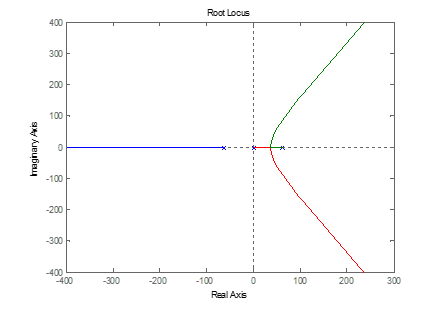Figure 1: Root Locus Plot of System Without Controller

When designing a controller, we only considered the transfer function between voltage and displacement, since voltage is the only input we have control over.  The transfer function between force and displacement cannot be controlled, since external force is a disturbance input.

We first graphed the root locus plot of the system without any control (Figure 1).  We can see that there are three poles and no zeros.  This means that there are three asymptotes, including two that will head into the positive real part of the graph.  Even worse, we see that the center of the asymptotes is located around zero, meaning that two of the poles will always be in the positive real part of the graph, and the system cannot be stable.

We know that we want a controller with zero steady state error, since the maglev train must be positioned with great accuracy, even a small steady state error is unacceptable.  This means that we need an integrator on the system.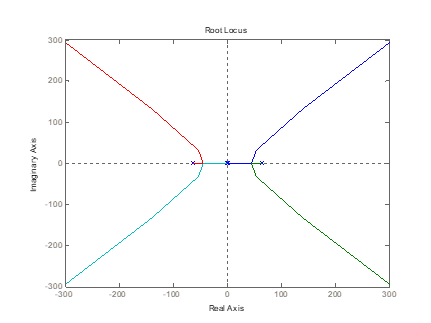Figure 3: Root Locus Plot of System With Integral Control

With an integrator, the system has four asymptotes, centered around zero (Figure 3).  Again, the system is unstable, and cannot ever be stable.  In order to make the system stable, we needed a way to move the center of the asymptotes over into the negative real plane, so that for at least some gain values, the system would be stable.  The best way to do this is derivative control.  With an ideal derivative controller, this is easy to accomplish.  We simply stick a few derivative controllers in the negative real plane, and the center of the asymptotes is moved.  As an added benefit, the number of asymptotes is lowered, so it is possible to create a system with only one asymptote, which will be stable forever.

The ideal derivative controller we used was Cderivative = s + 75.  In order to get a system degree of 1, we used three derivative controllers:

This system is completely stable for gain values that are sufficiently high.  There is a problem with it, however.  Ideal derivative controllers are not possible to create for a real life physical system, since they can create unrealistically large voltage spikes.  Also, derivative controllers are very sensitive to noise.  In order to create a realizable controller, we had to use a different kind of derivative controller, called a derivative gain limitation controller.

The derivative gain limitation controller contains both a pole and a zero.  The pole has a much greater magnitude than the zero, which means it does not affect the behavior of the controller much when compared to an ideal derivative controller.  However, the pole serves to limit the derivative controller’s maximum gain, as well as limiting the high frequency response of the controller, which helps to minimize the effect of noise (see Figure 4 for Bode plot comparison of the two types of controller).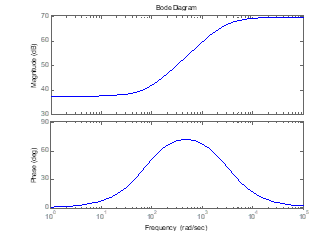Figure 5: Comparison of Ideal Derivative Controller (top) to Derivative Gain Limitation Controller (bottom)

We substituted our derivative gain limitation controller in for our derivative controller, and got the following root locus plot:Figure 6: Root Locus and Step Response of Stable System

This system finally has a stable solution.  We selected a gain that put all of the closed loop poles in the negative real plane, and tried the step response.  We found that the system’s overshoot was higher than our design specifications, meaning that the train would bob excessively when force is applied, and might even hit the track, which would be very bad.  In order to lower the overshoot, we have to increase the damping.

To increase the damping, we added a lead compensator to our system.  We based this choice on the bode plot of our system which showed that our phase margin was smaller than we wanted it to be (we wanted 21 degrees, see Figure 7).

We added the lead compensator, giving us our final controller design.Figure 8: Root Locus Plot of Controller (top) and Detail View of Origin (bottom)

Using MATLAB’s rlocfind()  and sgrid() functions, we found a gain that made the system stable, and was in the specifications we decided upon for the system.

Our final transfer function for the controller is:

We plotted the step response of the system: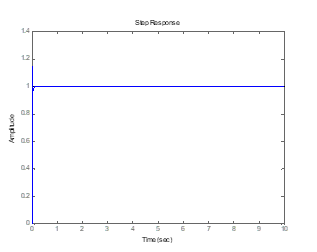Figure 9: Step Response of System with Final Controller for 0.1 Seconds (top) and 10 Seconds (bottom)

The overshoot is 38%, and the settling time is around 0.03 seconds.  These both fall below our controller goals of 50% overshoot and 0.1 second settling time.

The Bode plots for the system are as follows:Figure 10: Bode Plot of System with Controller (top) and Controller Alone (bottom)

# Sensors and actuators

The controller system needs a defined input to succeed his instruction. Therefore an accurate measurement of the current distance between trail and train is needed. One way to ensure this kind of measurement may be an optical method. An optical sensor like a laser or an infrared sensor would be an easy way to get current measurements during use of the maglev train. Certainly optical methods wear a kind of problems with it as impreciseness or susceptibility to noise, nevertheless they would succeed. These sensors are already used in maglev trains.

The disadvantages of an optical method lead to other kinds of sensors. Maglev trains, like the German “Transrapid”, use sometimes a kind of inductive measurements to calculate the distance between train and trail. This sensor uses the inductive forces in coins to determine the distance and to get an input for the controller system.

The actuator will be an amplifier with the digital controller attached. By the high susceptibility of the system the actuator should need an amplifier with a large range. The reaction of the digital controller has to be adequately to the amplified input.

# Works Cited

1. Downforce. (2008, September 11). In Wikipedia, The Free Encyclopedia. Retrieved 20:16, October 4, 2008, from http://en.wikipedia.org/w/index.php?title=Downforce&oldid=237824102
2. Electromagnet. (2008, October 4). In Wikipedia, The Free Encyclopedia. Retrieved 20:17, October 4, 2008, from http://en.wikipedia.org/w/index.php?title=Electromagnet&oldid=243008360
3. Elert, Glenn. “Inductance.” Hypertextbook. 1998. Hypertextbook. 28 Sept. 2008 <http://hypertextbook.com/physics/electricity/inductance/>. More details on calculating inductance.
4. Kuphaldt, Tony R. “Lessons in ElectricCircuits.” Lessons In Electric Circuits. 25 July 2007. Ibiblio. 28 Sept. 2008 <http://www.ibiblio.org/kuphaldt/electriccircuits/ac/>. We used pictures from this site for our resistor and inductor diagrams.
5. Maglev (transport). (2008, October 4). In Wikipedia, The Free Encyclopedia. Retrieved 20:16, October 4, 2008, from http://en.wikipedia.org/w/index.php?title=Maglev_(transport)&oldid=242899097
6. Magnetic levitation. (2008, October 2). In Wikipedia, The Free Encyclopedia. Retrieved 20:16, October 4, 2008, from http://en.wikipedia.org/w/index.php?title=Magnetic_levitation&oldid=242575897
7. NDT. “Self-Inductance and Inductive Reactance.” NDT Resource Center. NDT. 27 Sept. 2008 <http://www.ndt-ed.org/educationresources/communitycollege/eddycurrents/physics/selfinductance.htm>.
8. Pollard, M. G. “Maglev – A British First at Birmingham.” Physics Technology 15 (1984): 61-72. This paper was one of the few we found that actually had some technical info on maglev trains. Unfortunately, these trains were smaller models with the capacity for half a dozen people, but they gave us a data point to interpolate from.
9. PowerStream Technology. “Wire Gauge and Current Limits.” Wire Gauge and Current Limits. PowerStream Technology. 28 Sept. 2008 <http://www.powerstream.com/wire_size.htm>. Got our wire specifications here.
10. RailFanEurope. “TGV Dimensions.” TGV Web. Rail Fan Europe. 30 Sept. 2008 <http://www.railfaneurope.net/tgv/dimensions.html>. This is where we got dimensions for our train.
11. Vischer, D., and H. Bleuler. “Self-Sensing Active Magnetic Levitation.” IEEE Traqnsactions on Magnetics 29 (1993): 1276-281. We used this paper primarily for helping us understand the equations needed for our control system.
12. http://www.hochleistungsbahnen.tu-dresden.de/fachtagung_tr/trt5/12_trt5_vortrag12.pdf
13. “Index of /fachtagung_tr/trt5.”Kompetenzzentrum für Hochleistungsbahnen und Magnetbahnsysteme der TU . 5 Oct. 2008 <http://www.hochleistungsbahnen.tu-dresden.de/fachtagung_tr/trt5>.
14. “International Maglev Board.”International Maglev Board :: Index. 5 Oct. 2008 <http://magnetbahnforum.de/index.php?en_facts>.
15. “Transrapid International.”Transrapid International. 5 Oct. 2008 <http://www.transrapid.de/cgi-tdb/de/basics.prg?session=60ecdbba48e90feb_814119
16. “BBC NEWS | Business | Germany to build maglev railway.” BBC NEWS | News Front Page. 29 Oct. 2008 <http://news.bbc.co.uk/1/low/busin
17. “Derivative Control.” Welcome to 20-sim, the software for modeling dynamic systems. 3 Dec. 2008 <http://www.20sim.com/webhelp/library/signal/control/pid_control/derivativecontrol.htm>.
18. (WO/2006/079583) DISTANCE SENSOR ARRANGEMENT FOR A MAGNET OF THE LEVITATION MAGNET OF A MAGNETIC LEVITATION TRANSPORT SYSTEM.” WIPO – World Intellectual Property Organization. 3 Dec. 2008 <http://www.wipo.int/pctdb/en/wo.jsp?IA=EP2006050149&DISPLAY=DESC>.

This site uses Akismet to reduce spam. Learn how your comment data is processed.